OriMaths:: Main:: Introduction:: My own Designs:: Symbols:: OriMaths:: LinksA small game for beginners.

A small application of Origami in Mathematics, particularly in Plane Geometry will be shown here.

The results will seem to be obvious; however the steps are very attractive for beginners wether in Origami or Plane Geometry, since they will appear to be magical!

It's possible to execute them, e. g., in a school lesson due to its simplicity.

Because Origami involves paper and hands movement, this introduction will be very interactive - you will have to fold paper!

The surprise coefficient (!) will be a lot higher if you execute all tasks as you read on...

"Curiosity killed the cat" - this saying is also applied here. If you read everything without executing any of the simple tasks, all enthusiasm will die instantaneously.

The working materials are plain square shaped paper sheets.

How to obtain them? easy...

But first let's take a quick look over some symbols

 Symbols Symbol Meaning Example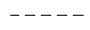Valley Fold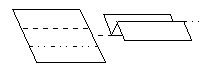Mountain Fold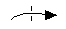Forward Fold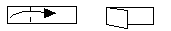Backward Fold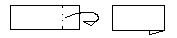Sink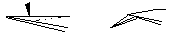Cut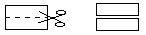The square is part of the rectangle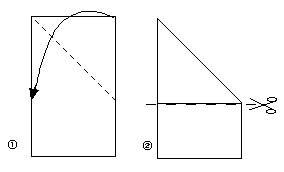The square has a width a and an area of - a2.

Let's begin:

First fold the square in half, transversally.Result: a square can be decomposed in two equal rectangles, each with sides of a e a/2.

But... there is another way to fold the square in half; which one? How about diagonally?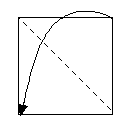Result: a square can be decomposed in two triangles, with cathetes of side a.

The rectangle obtained first and the triangle obtained later have both the same area! It doesn't look like it, does it?

Conclusion: a square can be decomposed in two equal rectangules, or two equal triangles, all with the same area!

Let us then go a bit farther!
(This was only the basics)

If possible, use two square shaped sheets of different colors to notice the differences and the similarities well.

Fold one of the sheets, generating the flower base, as it is shown in the next picture.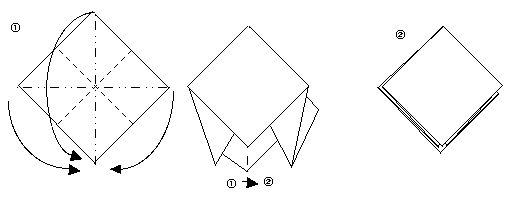How many squares do you count on the outside? Which dimensions have they?

Conclusion: a square can be put together by four other smaller squares, each one with a width a/2 and an area (a2)/4 relatively to the parent square.

Now fold the other sheet, generating the water bomb as shown next.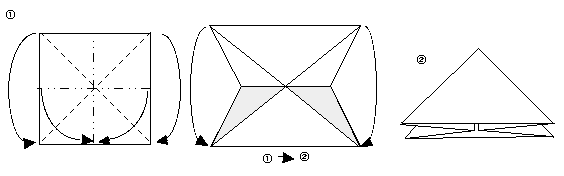Have you already counted the triangles? Which are its dimensions?

Conclusion: a square can be also formed by four triangles, each one with one hipotenusa of width a (what is the width of the cathets?) and an area of (a2)/4 relatively to the parent square.

Due to optic illusions, the second base seems to be "bigger" than the first one, right? But they equal themselves.

They are magically the reverse of each other! ...what?!

That is, you can transform a square, decomposed in triangles, in one which is decomposed in smaller squares, and vice versa, without applying any type of cuts! Wow!!

Each one of the mini-units will give another inverted mini-unit! (more precisely, each half unit of each two mini-units will give its reverse one) - complicated? Wait to see...

Let's do one more example to freshen up your mind

How do you do it? Easy!
Pick one of the bases, and sink the upper peak, as shown next, until...

..::: P O P ! ! :::..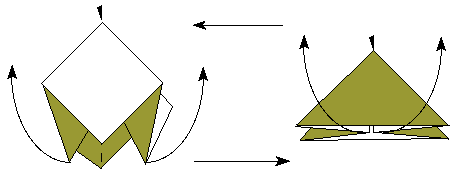...magically the other base comes out!

(Tip: while you pull the opposing borders out and up with the forefingers, push the peak with your thumb in the other direction!)

But are the bases equal to each other, or not?
t's easy to compare: put one inside the other!

Ending Moral:
You obtain proof of two different ideias, using the same process! Initially, you walk in opposing directions, but, at the end, you arrive at the same result!

These are the kind of challenges which attract Mathematicians!

 :: Main :: Introduction :: My Designs :: Symbols :: OriMaths :: Links
 Content: © v2.0 Rudolf Appelt